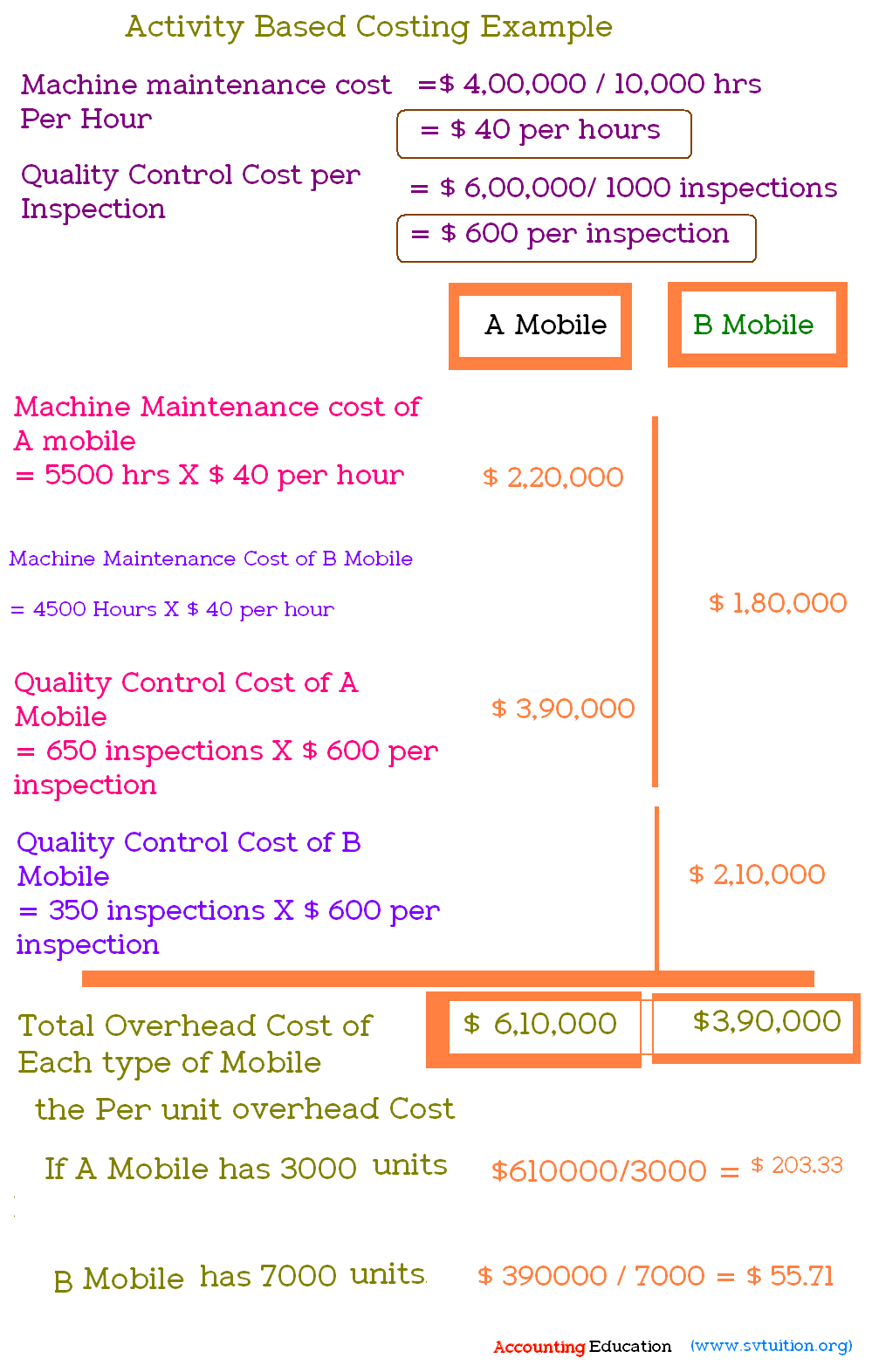Activity based costing is one of method of costing which is used for finding the exact cost of any product because in this method, we calculate the overhead cost on the basis of each activity on each product. With following example, you can understand it.

For example, Samsung mobile company is producing two type of mobile. One is A mobile and second is B mobile. Machine maintenance cost is \$ 4,00,000 and quality control cost is \$ 6,00,000. For producing both type of mobile, total machine hours spent 10,000 Machine hours (5500 hours spent on A mobile and 4500 hours spent on B mobile). For producing both type of mobile, total inspections are 1000. Samsung is producing A mobile's 3000 unit and B mobile's 7000 units.

If this company will use old method of calculating per unit cost, then, Total overhead cost is \$ 4,00000+\$ 6,00,000 = \$ 10,00,000. If we divide this overhead cost with total units, we will have per unit cost it will be

\$ 10,00,000 / 10,000 = \$ 100 per unit.

Important : It is not exact cost because we do not care which overhead spend on what mobile. So, we will take care each activity. See following screenshot.

From above screenshot, you will find that Mobile A's per unit cost is \$ 203 which is more than average cost per unit \$ 100. Second Mobile B's per unit cost is \$ 55.71 which is less than \$ 100 average per unit cost.

Important : Activity based costing is 100 times better than traditional method of finding cost per unit because it is so close to exact cost of each unit's cost. With this, we can fix correct sale price. For example, B mobile's overhead cost is so low as per activity based costing, so, we will fix less sale price of this product. A mobile's cost per unit is high, so, we will fix more sale price of this product. Except this, if  we have correct per unit cost, we can take many other good decisions like whether we have to continue any product or not.Comments on this Website: 2
.

## \$type=three\$a=hide\$cm=hide\$s=hide\$show=/2019/06/10-steps-to-become-better-investor.html\$l=hide

Name

false
ltr
item
Accounting Education: Activity Based Costing Example
Activity Based Costing Example
http://4.bp.blogspot.com/-muirBPJFZs4/VPgXY1aKkpI/AAAAAAAARRE/AFHx2FXL5Gw/s1600/activity%2Bbased%2Bcosting%2Bexample.PNG
http://4.bp.blogspot.com/-muirBPJFZs4/VPgXY1aKkpI/AAAAAAAARRE/AFHx2FXL5Gw/s72-c/activity%2Bbased%2Bcosting%2Bexample.PNG
Accounting Education
https://www.svtuition.org/2015/03/activity-based-costing-example.html
https://www.svtuition.org/
http://www.svtuition.org/
http://www.svtuition.org/2015/03/activity-based-costing-example.html
true
2410664366776677676
UTF-8